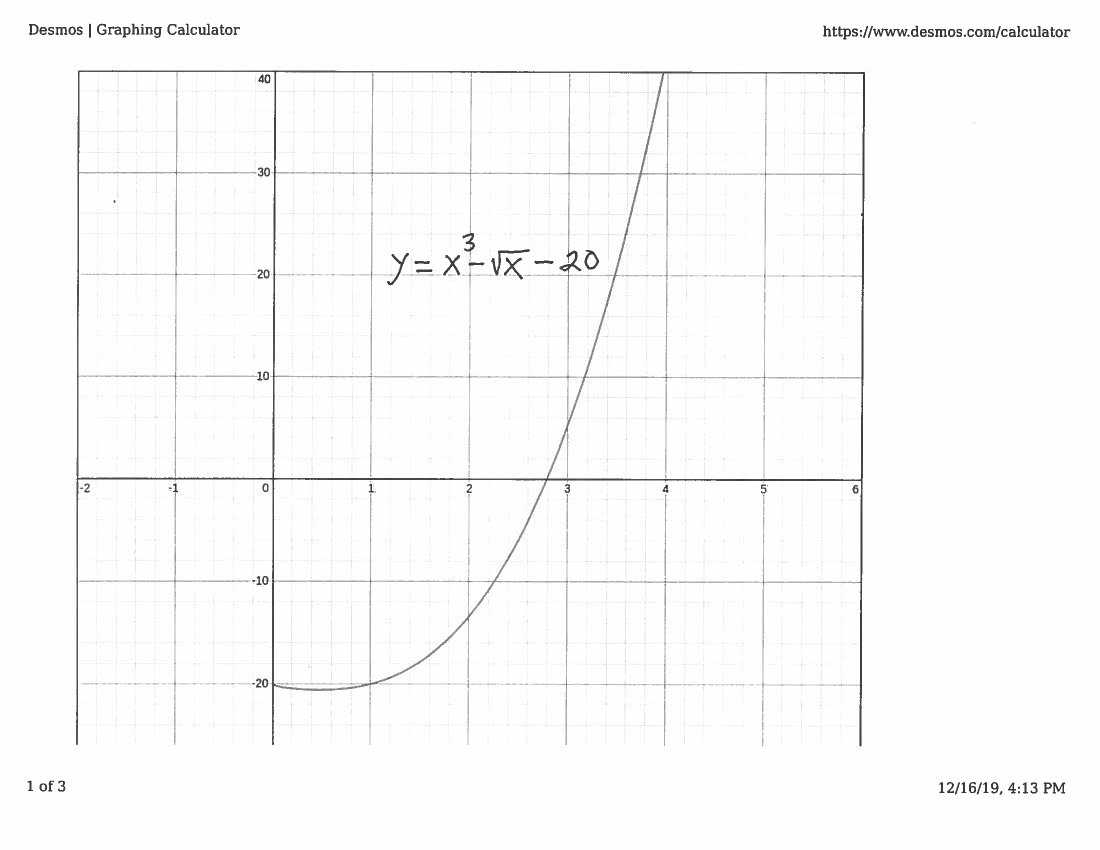SOLUTION 6: We are given the equation $$x^3=20+ \sqrt{x} \ \ \ \ \longrightarrow \ \ \ \ x^3-\sqrt{x}-20=0$$ Let function $$f(x)=x^3-\sqrt{x}-20 \ \ \ \ and \ choose \ \ \ \ m=0$$ This function is continuous for all values of $x \ge 0$ since it is the DIFFERENCE of continuous functions; it is well-know that $\sqrt{x}$ is continuous for all $x \ge 0$ and $x^3-20$ is continuous for all values of $x$ since it is a polynomial. To establish an appropriate interval consider the graph of this function. (Please note that the graph of the function is not necessary for a valid proof, but the graph will help us understand how to use the Intermediate Value Theorem. On many subsequent problems, we will solve the problem without using the "luxury" of a graph.)Note that $$f(1)= (1)^3-\sqrt{1}-20 =-20<0 \ \ \ \ and \ \ \ \ f(4)= (4)^3-\sqrt{4}-20=42>0$$
so that $$f(1)=-20 < m <42=f(4)$$
i.e., $m=0$ is between $f(1)$ and $f(4)$. Now choose the interval to be $\ [1, 4]$.

The assumptions of the Intermediate Value Theorem have now been met, so we can conclude that there is some number $c$ in the interval $[1, 4]$ which satisfies $$f(c)=m$$ i.e., $$c^3-\sqrt{c}-20=0$$ and the equation is solvable.